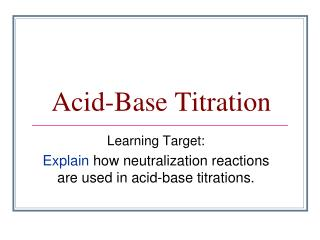DownloadDownload PresentationAcid-Base Titration

# Acid-Base Titration

Télécharger la présentation## Acid-Base Titration

- - - - - - - - - - - - - - - - - - - - - - - - - - - E N D - - - - - - - - - - - - - - - - - - - - - - - - - - -
##### Presentation Transcript

1. Acid-Base Titration Learning Target: Explain how neutralization reactions are used in acid-base titrations.

2. A neutralization reactionis a reaction in which an acid and a base in an aqueous solution react to produce a salt and water. • A salt is an ionic compound made up of a cation from a base and an anion from an acid. • Neutralization is a double-replacement reaction.

3. Acid Base Titration • Titrationis a method for determining the concentration of a solution by reacting a known volume of that solution with a solution of known concentration. • In a titration procedure, a measured volume of an acid or base of unknown concentration is placed in a beaker or flask, and initial pH recorded.

4. The standard solution is filled in a burette. • A couple drops of acid base indicator are added to the flask. • The standard solution is slowly added to the unknown solution in the flask. • As the two solutions are mixed the acid and the base are neutralized.

5. Standard Solution or titrant– An acid/ base solution whose concentration is known. • End point: The point at which an indicator changes color. • Equivalence point: The point at which moles of H+ ion from the acid equals moles of OH– ion from the base. An abrupt change in pH occurs at the equivalence point.

6. Titration of Strong acid & strong base • Since the salt produced is neutral, the solution at the equivalence point has a pH of 7. • the pH starts off low and increases as you add more sodium hydroxide solution. • Again, the pH doesn't change very much until you get close to the equivalence point. Then it surges upwards very steeply

7. Titration of weak acid and strong base • Salt is basic so, equivalence point comes at a pH > 7. • The start of the graph shows a relatively rapid rise in pH but this slows down

8. Titration of weak base & strong acid • Salt formed is acidic, hence, equivalence point comes at a pH < 7. • At the very beginning of the curve, the pH starts by falling quite quickly as the acid is added, but the curve very soon gets less steep.

9. Chemical dyes whose color are affected by acidic and basic solutions are called acid-base indicators.

10. Example… • A 43.0 mL of sodium hydroxide was titrated against 32.0 mL of 0.100 M hydrochloric acid. What is the molarity of sodium hydroxide solution?

11. Answer… • Step 1: Find the moles of hydrochloric acid: • Mol = M x L • Mol = 0.100 mol x .032 L L HCl = .0032 mol

12. Answer… • Step 2: Write a balanced equation, and find the molar ratio between the acid and the base. • HCl + NaOH  NaCl + H2O • Ratio:- HCl : NaOH 1 mol : 1mol • NaoH = 0.0032 Mol

13. Answer …. • Step 3: Find the concentration of sodium hydroxide. • M (NaOH) = mol/ L • M = 0.0032 mol .043 L • NaOH = 0.0744 M